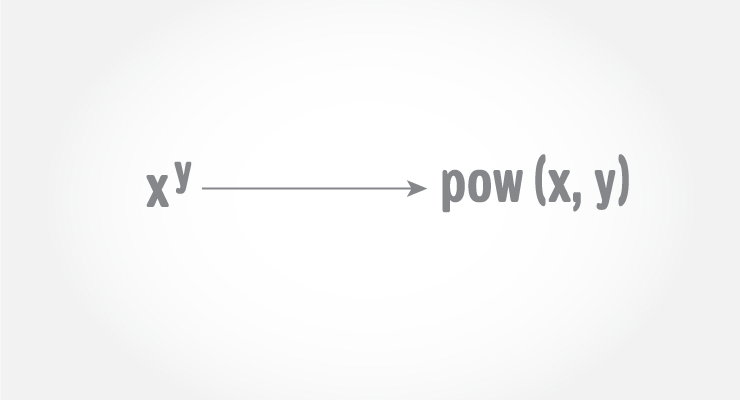# C pow()

The pow() function computes the power of a number.The pow() function takes two arguments (base value and power value) and, returns the power raised to the base number. For example,

`[Mathematics] xy = pow(x, y) [In programming]`

The `pow()` function is defined in `math.h` header file.

## C pow() Prototype

`double pow(double x, double y)`

The first argument is a base value and second argument is a power raised to the base value.

To find the power of int or a float variable, you can explicitly convert the type to double using cast operator.

```int base = 3;
int power = 5;
pow(double(base), double(power));```

## Example: C pow() function

``````#include <stdio.h>
#include <math.h>

int main()
{
double base, power, result;

printf("Enter the base number: ");
scanf("%lf", &base);

printf("Enter the power raised: ");
scanf("%lf",&power);

result = pow(base,power);

printf("%.1lf^%.1lf = %.2lf", base, power, result);

return 0;
}``````

Output

```Enter the base number: 2.5
Enter the power raised: 3.4
2.5^3.4 = 22.54```